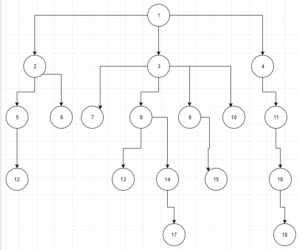# Java Concurrency using Fork/Join Framework

The Fork/Join Model is a parallel design pattern that sets up and executes concurrently code parts. The execution divides in parallel at multiple points in the program, performs a task in parallel and later it joins at a single point.

In java it is implemented by the Fork/Join Framework, an implementation of ExecutorService interface, that help us take advantage of multiple processors by splitting the tasks into smaller pieces and execute them in parallel. That helps us enhance the performance of our application.

Usually we use this framework in cases that we have heavy jobs that cause delays and can be broken into smaller tasks. One use case that I have faced in the past is the tree parsing. Let’s assume that we need to parse a tree and perform some costly actions (algorithms, database queries) for every node we visit. If the tree is big enough the execution could take hours, or even days.

By using a divide and conquer approach, with the help of the fork/join framework, we will show how we can drastically reduce the execution time.

The full github repository can be found here: fork-join-tree

## Technologies Used

• Java
• Spring Boot
• Design Patterns
• Fork/Join
• Concurrency

The problem

We want to parse the following tree :As we can see in the diagram, each node can have one, many or none children.

We assume that for each node that we visit we need to perform some time consuming actions. We will simulate that by using the Thread.sleep() method.

It is clear that if we visit each node sequentially the duration for the complete tree parsing will be long.

Preparation

The first thing that we need to do is to construct the problem in order to solve it. The next pojo class illustrates the tree.

Node.js

```public class Node<T> {
private List<Node<T>> children;
private T data;
public Node(T data, List<Node<T>> children) {
this.children = children;
this.data = data;
}
public List<Node<T>> getChildren() {
return children;
}
public T getData() {
return data;
}
}```

In this class we have two variables the children of the current node and the data that it contains. The children variable is a list of other nodes, and each one of them has its own children itself.

Next we need to construct the tree. We will use the following class for this. We need to configure the application.properties file with the correct path for our text file which contains the tree structure. We use the variable tree.file.path for that. After that the readTree() method loops the text lines and create a node for each one of them.

TreeService.java

```@Service
public class TreeService {

@Value("\${tree.file.path}")
private String treeTxtPath;

public Node<String> getTree(String nodeName){
return treeMap.get(nodeName);
}

Map<String, Node<String>> nodes = new HashMap<>();
String line, nodeName, words[];
List<Node<String>> children;
while ((line = br.readLine()) != null){
children = new ArrayList<>();
words = line.split(" ");
nodeName = words;
for (int i = 1; i < words.length; i++) {
}
nodes.put(nodeName, new Node<>(nodeName, children));
}
} catch (IOException e){
e.printStackTrace();
}
return nodes;
}
}```

Normal tree parsing

First we will parse the created tree normally, without the fork/join pattern. We use a recursive method for that, which gets all the children of the current node and calls itself for each one of them. Also we force the Thread to sleep using the sleep method. We are doing that to simulate the task’s action that takes much time. This could be some complicated algorithmic calculations on big data, an external call or a database query.

ParseService.java

```public void parseTree(Node<String> node) {
for(Node<String> child : node.getChildren()){
parseTree(child);
try {
} catch (InterruptedException e) {
e.printStackTrace();
}
}
System.out.print(node.getData() + " ");
}```

Parallel tree parsing

We add the current node in the nodes list. As we did on the normal tree parsing we will again simulate a delay using Thread’s sleep() method.

After that we loop the node’s children and for each one we fork a new task. By doing this we are able to drill down the whole tree.

The final step is to join all the tasks using the join() method. Finally we return the Node object which contains the whole tree.

```public class ParseTreeTask extends RecursiveTask<List<Node<String>>>{

private final Node<String> currentNode;

this.currentNode = currentNode;
}

@Override
protected List<Node<String>> compute() {
List<Node<String>> nodes = new ArrayList<>();
try {
} catch (InterruptedException e) {
e.printStackTrace();
}
if(currentNode.getChildren() != null)  {
for(Node<String> child : currentNode.getChildren()){
}
}
}
return nodes;
}
}```

Now that we have prepared the task, all we need to do is to call it. In our service method we create a new ForkJoinPool and we set the parallelism number (in our case 15). After that we create and new ParseTreeTask and we pass the root object. Then we invoke it using the ForkJoinPool object.

After using the ForkJoinPool we need to close it. We perform the closing action in the finally block to ensure that it will run in any case.

ParseService.java

```public void parseTreeInParallel(Node<String> node){
final ForkJoinPool forkJoinPool = new ForkJoinPool(15);
List<Node<String>> tree = new ArrayList<>();
try {
} catch (final Exception e){
forkJoinPool.shutdownNow();
e.printStackTrace();
} finally {
forkJoinPool.shutdown();
}
printTree(tree.get(0));
}

private static void printTree(Node<String> node){
for(Node<String> child : node.getChildren()){
printTree(child);
}
System.out.print(node.getData() + " ");
}```

Results

Using mvn spring-boot:run we run the application and check the logs.

We notice that the normal tree parsing lasted for 17008 milliseconds. After that the fork/join tree parsing  ran which lasted 5027 milliseconds.

```12 5 6 2 7 13 17 14 8 15 9 10 3 18 16 11 4 1
Duration without parallelization : 17008
12 5 6 2 7 13 17 14 8 15 9 10 3 18 16 11 4 1
Duration with parallelization : 5027```

Setup and Run

• Update the application.properties file with the tree.txt path on your machine.
• mvn spring-boot:run